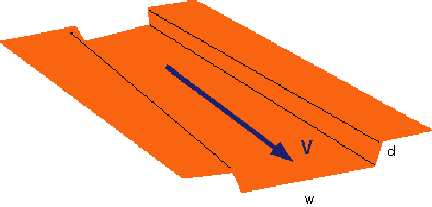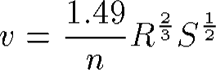## Assignment 10: River Discharge Function

River discharge function

The volume of water that passes by a point in a river over a certain time increment is the discharge (for example, the Tempe Town Lake can apparently withstand about 40,000 cubic feet per second with the dams up). The discharge can be simply expressed as the product of the velocity times the cross-sectional area of the flow:
Q = v * A, where Q is discharge [L^3/T], v is velocity [L/T], and A is area [L^2]
Many channels are effectively rectangular, so we can express the cross-sectional area as the product of width (w) times depth (d):
A = w * dSo how to calculate the velocity?
We use a simple formulation called Manning's equation that relates the flow velocity to the local channel slope (s [dimensionless]), a channel shape parameter called the hydraulic radius [L]--see below, and a roughness parameter n [L^1/6]. NOTE THAT THIS IS FOR ENGLISH UNITS (feet, seconds):The hydraulic radius R is equal to the ratio of the channel area (A) to its wetted perimeter (P):
P = w + 2d
R = A/P
The Manning roughness coefficient (n) is estimated based upon inspection of the channel and its shape. Here is a table of roughnesses depending on the channel boundary type.

Here is a link with similar explanation and a few pictures and hydrographs from 2005 flow along the Salt River: pdf. A basic video explanation of the MATLAB implementation of these equations is here:

For this homework, I would like for you all to build a couple of functions that make some interesting geomorphic calculations. Build a web page for this assignment and present your results and answers to the following set of questions:

1) Develop a well documented Matlab function that will calculate the velocity and the discharge at a point given the channel shape, roughness, and slope parameters. Make sure that you use the fprintf command to write the variables back to the command line and let the user know what is going on.
2) Make a plot of the relationship of velocity versus channel slope for values of slope varying from 0 to 0.2 (about 12 degrees), all other variables constant. How does velocity vary with channel slope?
3) Make a plot of the relationship of discharge versus channel slope for values of slope varying from 0 to 0.2 (about 12 degrees), all other variables constant. How does discharge vary with channel slope?
4) Make a plot of the relationship between velocity and hydraulic radius, all other variables constant. Choose a range of radius values that are for shallow and deep channels. All things being equal, is the velocity faster in a deeper or shallower channel?
5) Make a plot of the relationship between velocity and channel roughness, all other variables constant. Vary the roughness from that of a smooth glass chute to a mountain stream. How does the velocity vary with roughness?
6) Make a plot of the relationship between discharge and channel roughness, all other variables constant. Vary the roughness from that of a smooth glass chute to a mountain stream. How does the discharge vary with roughness?
7) If you were to be watching the Salt River at the downstream end of the Tempe Town Lake and the water were flowing across the location of the downstream dam that is 19' high and the channel width is about 850' and the channel slope there is 0.0013 (Arrowsmith actually calculated based on surveyed elevations in the channel), what would the flow velocity and discharge be (you estimate the roughness)?
8) If you were watching the Salt River at the downstream end of Tempe Town Lake and the water were bank full (to the top of the levees=40'), the channel width were 900' and the slope was the same as above, what would the discharge and velocity be (you estimate the roughness)? In 1993, the river was supposedly flowing at 100,000 cubic feet per second. Based upon your calculations, was it at bankfull?

• M-files: (15 points)
• Function for calculating velocity and discharge
• Script for plotting and calculations
• Five Plots (5 points each)
• #7, include justification of roughness coefficient based on inspection (5 points)
• #8, include justification of roughness coefficient based on inspection (5 points)

Assignment is due Wednesday, October 21, 2015 before class .